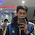Electromagnetic Induction

The process, by which a changing magnetic field in a conductor induces a current in it, is called Electromagnetic Induction.

Activity 1

1.    Take a coil of wire having many turns.
2.     Connect it to a sensitive galvanometer.
3.     Take a bar magnet and move the north pole of the magnet towards the coil.Observation: you will observe that galvanometer will give a deflection in one direction (say left).

4.    Now move the north pole of the magnet away from the coil.

Observation: you will observe that galvanometer now give a deflection in opposite direction (now right)

**Similar effect will be observed if we use the south pole of the magnet in the above activity. But the direction of deflection will be reversed.

**Similar effect will be observed if we keep the magnet stationary and move the coil towards or away from the coil.

**When the coil and the magnet are both stationary, there is no deflection in the galvanometer.

Thus whenever there is a relative motion between the coil and the magnet, it induces a current in the coil.

Summary of the activity

 POSITION OF THE MAGNET DEFLECTION IN THE GALVANOMETER Magnet at rest No deflection in galvanometer Magnet moves towards the coil Deflection in galvanometer in one direction Magnet is held stationary at same position (near the coil) No deflection in galvanometer Magnet moves away from the coil Deflection in galvanometer but in opposite direction Magnet is held stationary at same position (away from the coil) No deflection in galvanometer Magnetic is held stationary inside the coil No deflection in galvanometer

Activity 2

1.    Take two different coils of copper wire having large number of turns (say 50 and 100 turns respectively). Insert them over a non-conducting cylindrical roll

2.    Connect the coil-1, having larger number of turns, in series with a battery and a plug key.
3.    Also connect the other coil-2 with a galvanometer as shown.
4.    Plug in the key. Observe the galvanometer.
Observation: You will observe that the needle of the galvanometer instantly jumps to one side and just as quickly returns to zero, indicating a momentary current in coil-2.
5.    Disconnect coil-1 from the battery.  Observe the galvanometer.

Observation: You will observe that the needle momentarily moves, but to the opposite side. It means that now the current flows in the opposite direction in coil-2.

Thus form activity 1 and activity 2 it is clear that we can induce current in a coil either by moving it in a magnetic field or by changing the magnetic field around it. It is convenient in most situations to move the coil in a magnetic field.

The induced current is found to be the highest when

the direction of motion of the coil is at right angles to the magnetic field. In this situation, we can use a simple rule, Fleming's Right Hand Rule, to know the direction of the induced current.

Fleming’s Right Hand Rule

Hold the forefinger, the central finger and the thumb of the right hand perpendicular to each other so that the forefinger indicates the direction of the field, and the thumb is in the direction of motion of the conductor. Then, the central finger shows the direction of the current induced in the conductor.

1.2.3.4.